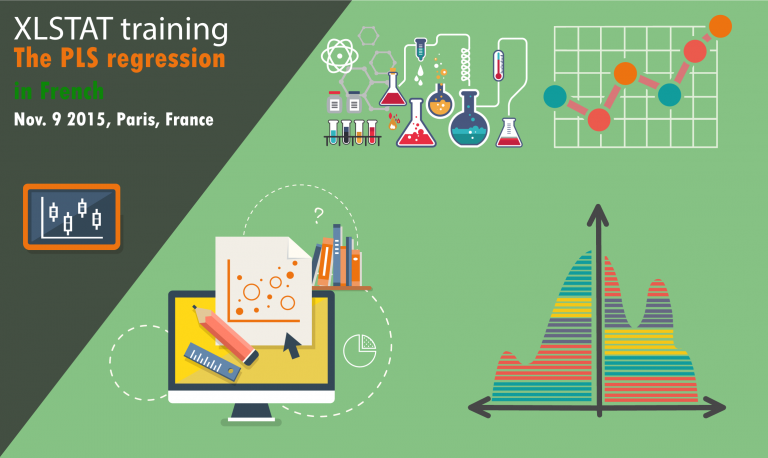# The PLS regression, 1 day training (in French)The Partial Least Square Regression (PLS regression) is a powerful statistical method that can be used in many situations where the classical regression is not applicable. For example, it can be used even if there is a strong multicollinearity in the data or if there are more variables than observations or if there are missing data. It is typically used in chemometrics or in OMICs data analysis. Subscribe to this training session that will let you understand precisely the principles of this method and learn how to use it in XLSTAT.

This session is over

#### The PLS regression, 1 day training

A training on the PLS (Partial Least Square) regression that works in situations where classical regression techniques cannot be applied, for example when there is strong multicollinearity in your data, or if you have more variables than observations or if there are missing data. The training is followed by practical application exercises and tests to be submitted online for correction.

Prerequisites

Basic knowledge in statistics

Objectives

• Understand the PLS regression principles
• Learn when to apply the PLS regression
• Learn how to use the XLSTAT software to apply a PLS regression
• Learn how to read and interpret PLS regression outputs

Training program

• Introduction to the PLS approach
• Reminder on classical linear regression
• The principal component regression
• The PLS1 regression
• The PLS2 regression
• The PLS discriminant analysis
• Applications on real data

Company/Private

€450.00
per participant

Start at:

End at:

French

Paris France

#### Emmanuel Jakobowicz

##### Founder of Stat4decision

With more than 15 years of experience in data-oriented projects, Emmanuel Jakobowicz is passionate about data science and entrepreneurship. He founded Stat4decision to propose a new way of assisting people in exploring their data. He owns a PhD in applied statistics and computer science. He also is an engineer in mathematics specialized in machine learning. His work experience includes research and development at Electricité de France and software development at Addinsoft XLSTAT where he was a partner, CTO, Chief Scientist, consultant and trainer for large companies, research institutes and universities.